## Cipher## Jan 31, 2017

### CNN - Trump travel ban is 'flat anti-American' 227 318 once again

Earlier today I decoded a story with a lot of 318 153 227 connections and CNN released another post with these incredible numbers

CNN's article - http://edition.cnn.com/2017/01/31/politics/madeleine-albright-trump-travel-ban-anti-american-cnntv/index.html
If you are new to these numbers check the previous post first - CNN-318-153-bible-reference-with-lucy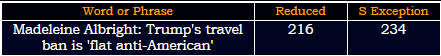216 = 6*6*6
234+432 = 666Madeleine = 4+1+4+5+3+5+9+5+5 = 41 (Reduced)
Albright = 1+3+2+9+9+7+8+2 = 41 (Reduced)
13th prime number is 41
The story comes out on 31st day of the year - 1/31 = 32 (32nd prime number is 131)

Her birthday is on 15th of May which is 104 days from nowFifteenth of May = 6+9+6+20+5+5+14+20+8 + 15+6 + 13+1+25 = 153 (Ordinal)
5/15 is 135th day in the year (135+531 = 666)
This story comes from New Day (CNN's TV show)
New day = 40+5+900 + 4+1+400 = 1350 (Jewish)
New day = 84+30+138 + 24+6+150 = 432 (Sumerian)
Their tweet shows 2:34 PM - 234+432 = 666
January Thirty first = 600+1+40+200+1+80+400 + 100+8+9+80+100+400 + 6+9+80+90+100 = 2304 (Jewish)

Madeleine = 30+1+4+5+20+5+9+40+5 = 119 (Jewish) - Saturn = 119 (Francis Bacon)
Albright = 1+20+2+80+9+7+8+100 = 227 (Jewish)
It's very interesting that she has these 2 numbers
119+191+911 = 1221
227+272+722 = 1221

Former Secretary of State Madeleine Albright is slamming President's Trump's travel ban as reckless and "flat anti-American."

"flat anti-American." = 6+3+1+2 + 1+5+2+9+1+4+5+9+9+3+1+5 = 66 (Reduced)
"We are a country that has been created and populated by people from other countries," she told CNN's Chris Cuomo on Tuesday
We are a country that has been created and populated by people from other countries, = 6006 (English)
Thirty three 66 (Reduced)

"We are a country that has been created and populated by people from other countries," = 318 (Reduced)

Just before they end the story they drop another 227 connecting to her last name
"Disruption is interesting, Chris, (but) destruction is bad," she said.

"Disruption is interesting, Chris, (but) destruction is bad," = 227 (Reduced)
Twenty two divided by seven = 314
Seven hundred seventy seven = 314"and there's going to be tit for tat." = 1+5+4 + 2+8+5+9+5+10 + 7+6+9+5+7 + 2+6 + 2+5 + 2+9+2 + 6+6+9 + 2+1+2 = 137 (S Exception)
33rd prime number is 137

Trump signed a 120 day ban "to keep the refugees from entering the country and to ban immigrants from seven predominantly Muslim nations"
one hundred twenty days = 6+5+5 + 8+3+5+4+9+5+4 + 2+5+5+5+2+7 + 4+1+7+1 = 93 (Reduced)
Note how in the bottom of their post there is a related video that is 2:26 minutes long
Two hundred twenty six = 2+5+6 + 8+3+5+4+9+5+4 + 2+5+5+5+2+7 + 1+9+6 = 93 (Reduced)
Saturn = 19+1+20+21+18+14 = 93 (Ordinal)

Also there's this - She said the sudden ban has "created more danger because there are countries that are now, in fact, not able to cooperate with us in terms of intelligence sharing."
"created more danger because there are countries that are now, in fact, not able to cooperate with us in terms of intelligence sharing." = 558 (S Exception)
Saturn = 114+6+120+126+108+84 = 558 (Sumerian)

### CNN 318 153 bible reference with Lucy and Saccorhytus "our earliest ancestor"

3.14 and 0.318 are special numbers related to the circumference of a circle
22/7 = 3.142857... is the closest approximation of Pi with small numbers (333/106 = 3.141509... or 355/113 = 3.1415929...)
7/22 = 0.318 which is sort of inverse Pi
You can calculate the circumference of any circle just by dividing 2x radius or diameter by 0.318

So if we decided for our circle to have a radius of 8 it would be (8x2)/0.318 = 50.3144654...
This picture is from online calculator that calculates the circumference like we are taught in school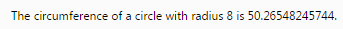318 is also one of important biblical numbersGenesis 14:14 is 351st verse in the Old Testament

The second coming of Jesus Christ is mentioned 318 in the New Testament
And the most important part is the miraculous catch of 153 fish in John 21:11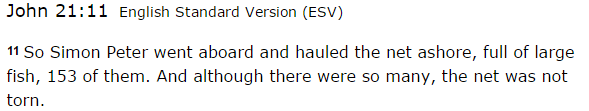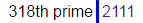21*11 = 231 (octal of 153)

Also 1122nd minute of the day leaves 318 minutes

These are the 2 CNN stories that are connected with 318 and 153
http://edition.cnn.com/2016/08/29/health/lucy-early-human-ancestor-cause-of-death/index.html
http://edition.cnn.com/2017/01/30/health/earliest-human-ancestor-deuterostome-saccorhytus-history-study/index.html

Yesterday on January 30th CNN released a story saying that microfossils from China have revealed our "earliest ancestor" which supposedly lived 540 million years ago and as you scroll through the story they have a related story on the left, which gave me the first clue, then I saw how they mention that Lucy our 'early ancestor' died 3.18 million years ago

This is how they showed related articleThis is from August 30th 2016 saying that Lucy provided us with new insight about our 'early ancestors' 3.18 million years agoFrom Lucy's article to January 30th is 153 days of course also it is 21 weeks and 6 days a lot like 216 (6x6x6)
Saturn = 19+1+20+21+18+14 = 93 (Ordinal)
Thirtieth of January = 2+8+9+9+2+9+5+2+8 + 6+6 + 1+1+5+3+1+9+7 = 93 (Reduced)three hundred eighteen = 2+8+9+5+5 + 8+3+5+4+9+5+4 + 5+9+7+8+2+5+5+5 = 113 (Reduced)
one hundred fifty three = 6+5+5 + 8+3+5+4+9+5+4 + 6+9+6+2+7 + 2+8+9+5+5 = 113 (Reduced)
one five three = 50+40+5 + 6+9+700+5 + 100+8+80+5+5 = 1013 (Jewish)
miraculous catch of fish = 4+9+9+1+3+3+3+6+3+10 + 3+1+2+3+8 + 6+6 + 6+9+10+8 = 113 (S Exception)
fish = 6+9+90+8 = 113 (Jewish)
Second coming of Jesus Christ = 1+5+3+6+5+4 + 3+6+4+9+5+7 + 6+6 + 1+5+1+3+1 + 3+8+9+9+1+2 = 113 (Reduced)
November 3rd is written 11/3 in some parts of the world
November Third = 14+15+22+5+13+2+5+18 + 20+8+9+18+4 = 153 (Ordinal)
Note that this story was released on 30th day of the yearSaturn = 10+1+2+3+9+5 = 30 (S Exception)
Saturn's orbital period is between 28-30 years (360 months - Three hundred sixty = 227)
Jesus Christ = 10+5+19+21+19 + 3+8+18+9+19+20 = 151 (Ordinal)It's all very interesting when you consider how 151 is 227 in octal system, 318th prime is the verse in which Jesus Christ catches 153 fish and we have "Anti-Pi" and Pi meeting there

Story about Lucy was released on a date with 74 numerology which even more corresponds to Jesus or Lucifer (74)
8/30/2016 - 8+30+20+16 = 74 (Jesus = 74) also Lucy was 'found' in 1974
Jesus is the son of 'God' = 7+6+4 =  17 - Reduced  (son of the God 'EL' = 5+12 = 17)
If you turn 7 you can get L and the letters E and L are in the same positions in English and in Hebrew alphabet
7th prime number is 17 which is the reason it has great significance and the 17th triangular number is 153
17th tetrahedral number is 969 (science says that speed of Saturn is 9.69km/s)
It's interesting that 107 converted into Octal system becomes 153
Also another number related to Saturn is 119 which is 7x17 also 156th prime number is 911
Saturn = 45+1+20+21+18+14 = 119 (Francis Bacon)
God Saturn = 7+15+4 + 19+1+20+21+18+14 = 119 (Ordinal)

Back to the story,  the cycle isn't complete with this story coming out today, from what I'm seeing they are pointing to the release of the new Alien movie on May 19th 2017
This is how they opened the post from today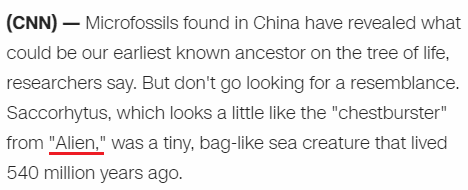There's many reasons this is interesting
May Nineteenth = 13+1+25 + 14+9+14+5+20+5+5+14+20+8 = 153 (Ordinal)
5/19/17 - 5+19+17 = 41
1/30/2017 - 1+30+2+0+1+7 = 41
Alien = 1+12+9+5+14 = 41 (Ordinal)
13th prime number is 41 and 13 is also the number related to Saturn
13th Fibonacci number is 233 which is also 351 in Octal numbering system
God = 7+15+4 = 26 - Ordinal (26th triangular number is 351)
(Something interesting I found today - 13th perfect number is 314 digits long)

The movie is called "Alien: Covenant" https://en.wikipedia.org/wiki/Alien:_Covenant
Alien: Covenant = 1+3+9+5+5 + 3+6+4+5+5+1+5+2 = 54 (Reduced)
They are saying that this creature lived 540 million years ago?
Alien: Covenant = 1+12+9+5+14 + 3+15+22+5+14+1+14+20 = 135 (Ordinal)
135+531 = 666They are giving a tribute on a date with 93 gematria to a date that leaves 226 days in the year and both are numerological match

Note that 318th day in the year is November
November Fourteenth = 14+15+22+5+13+2+5+18 + 6+15+21+18+20+5+5+14+20+8 = 226 (Ordinal)
Two hundred twenty-six = 2+5+6 + 8+3+5+4+9+5+4 + 2+5+5+5+2+7+1+9+6 = 93 (Reduced)
November Fourteenth = 40+50+700+5+30+2+5+80 + 6+50+200+80+100+5+5+40+100+8 = 1506 (Jewish)
One hundred fifty six = 15+14+5 + 8+21+14+4+18+5+4 + 6+9+6+20+25 + 19+9+24 = 226 (Ordinal)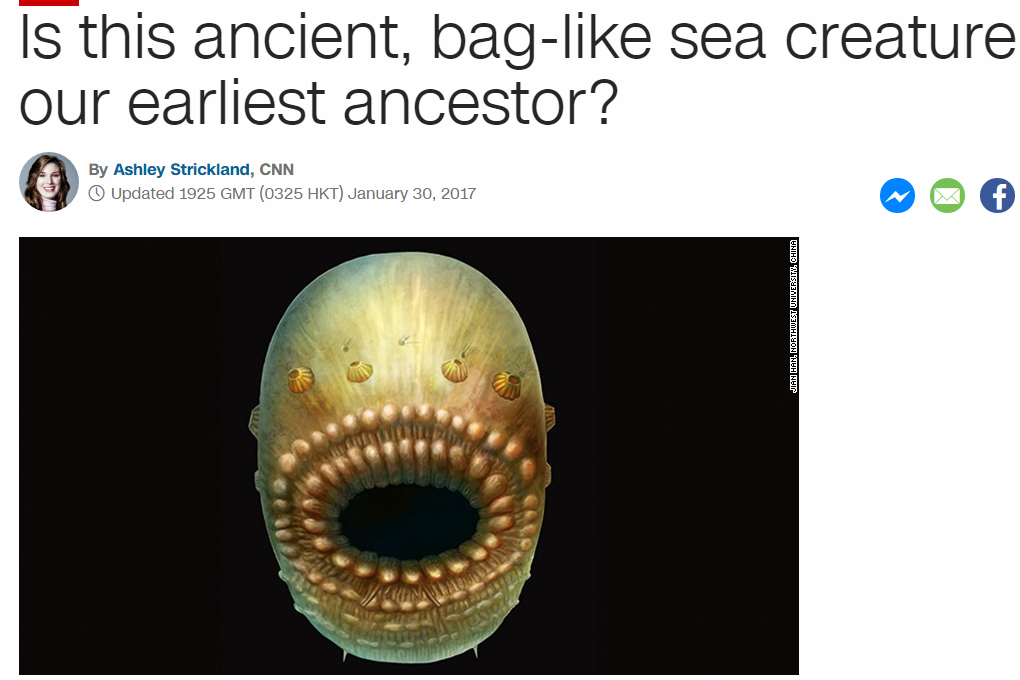They compare it to "Chestburster" from Alien
chestburster = 3+8+5+100+200+2+300+90+100+200+5+90 = 1103 (English)
Saccorhytus = 1+1+3+3+6+9+8+7+2+3+1 = 44 (Reduced)
They end their post with this
Funny to think, but in half a billion years, a lot of evolutionary changes can take place = 330 (S Exception)

Mithras = 30+9+100+8+80+1+90 = 318 (Jewish) - Roman Sun God before they adopted Christianity

From 11/14 (318th day) to 1/30/2017 is 77 days, which is interesting and shows why there's at least 3 stories today with 318 coding
Christ = 77 (7x7 = 49)
49th prime is 227 - Miraculous catch of fish - Pi (227) and "inverse Pi" (318) coding
Twenty-two divided by seven = 314 (Ordinal)
Also 3/18 is 77th day of the yearSome unrelated finds
314 in Roman numerals - CCCXIV = 3+3+3+6+9+4 = 28 (Reduced)
28 is 2nd perfect number also 7th triangular number is 28The number of Skull and Bones is 322 (322+232+223 = 777)
The number for Saturn in Jewish gematria is 511 (511+151+115 = 777)

Adding all combinations of 314 we arrive at 1776 the creation of Bavarian Illuminati and America's declaration of Independence
314+341+143+134+431+413 = 1776

## Jan 29, 2017

### People drifting in waters off Malaysian coast await rescue

CNN's post before any updates -  http://edition.cnn.com/2017/01/29/asia/malaysia-boat-missing-tourists/index.htmlPeople drifting in waters off Malaysian coast await rescue = 228 (Reduced)
Death = 24+30+6+120+48 = 228 (Sumerian)44th prime number is 193 (Kill = 44, Execution = 44)This story reminds me of the Malaysian story which is entirely coded out with crazy numbers, Malaysian airplane disappeared on 3/8/2014 with 227 passengers
227 is one of the special numbers, the airplane that disappeared was Boeing 777, 227 converted to Octal is 343 (7x7x7), it is also the number connected to Pi - 22/7 = 3.14 and both 227 and 314 are very frequently coded
The incident occurred during the Chinese New Year celebration period.The boat sank off coast of Sabah in Malaysia
coast of Sabah = 3+6+1+1+2 + 6+6 + 1+1+2+1+8 = 38 (Reduced)
Death = 4+5+1+20+8 = 38 (Ordinal)

Sabah = 1+1+2+1+8 = 13 (Reduced)
Sabah = 19+1+2+1+8 = 31 (Ordinal)
31 people were on the boat and the police report was lodged 13 hours later
The story comes on a date with 67 numerology (1+29+20+17 = 67)
Three hundred thirty one = 100+8+80+5+5 + 8+200+40+4+80+5+4 + 100+8+9+80+100+400 + 50+40+5 = 1331 (Jewish)
They were traveling on a catamaran
catamaran = 3+1+2+1+4+1+9+1+5 = 27 (Reduced)
Malaysia = 4+1+3+1+7+1+9+1 = 27 (Reduced)

Malaysian coast = 13+1+12+1+25+19+9+1+14 + 3+15+1+19+20 = 153 (Ordinal)
twenty eight Chinese tourists = 2+5+5+5+2+7 + 5+9+7+8+2 + 3+8+9+5+5+10+5 + 2+6+3+9+9+10+2+10 = 153 (S Exception)
28th prime number is 107 (107 converted to Octal is 153, 17th triangular number is 153)

The boat is believed to have sunk near Mengalum Island after it sailed from Kota Kinabalu, and two crew members managed to make their way to land to seek assistance, the report says.
Mengalum Island = 4+5+5+7+1+3+3+4 + 9+1+3+1+5+4 = 55 (Reduced)
Kota Kinabalu = 11+6+2+1 + 11+9+5+1+2+1+3+3 = 55 (K Exception)
two crew members = 7+4+2 + 3+5+5+4 + 1+5+1+2+5+5+6 = 55 (Septenary)
The Malaysian airplane debris were found on 7/29/2015 which is also 550 days until the release of this story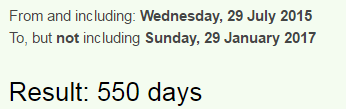Mengalum = 30+5+40+7+1+20+200+30 = 333 (Jewish)
Kota Kinabalu = 10+50+100+1 + 10+9+40+1+2+1+20+200 = 444 (Jewish)
Kota Kinabalu = 11+15+20+1 + 11+9+14+1+2+1+12+21 = 118 (Ordinal)
Death = 4+5+1+100+8 = 118 (Jewish)
The maritime agency enforcement agency, the navy and the air force were conducting the search, which was hampered by bad weather, Xinhua said.
Xinhua = 300+9+40+8+200+1 = 558 (Jewish)
Saturn = 114+6+120+126+108+84 = 558 (Sumerian)

Xinhua = 24+9+14+8+21+1 = 77 (Ordinal)
Judaism = 10+21+4+1+9+19+13 = 77 (Ordinal)
Christ = 3+8+18+9+19+20 = 77 (Ordinal)

There were 3 crewmen on the boat and 28 tourists
three crewmen = 20+8+18+5+5 + 3+18+5+23+13+5+14 = 137 (Ordinal)
33rd prime number is 137
twenty eight tourists = 2+5+5+5+2+7 + 5+9+7+8+2 + 2+6+3+9+9+1+2+1 = 90 (Reduced)
Ninety = 5+9+5+5+2+7 = 33 (Reduced)

Twenty eight = 20+23+5+14+20+25 + 5+9+7+8+20 = 156 (Ordinal)
Thirty three = 20+8+9+18+20+25 + 20+8+18+5+5 = 156 (Ordinal)

### Trump signs executive actions on lobbying, ISIS, NSC

http://edition.cnn.com/2017/01/28/politics/donald-trump-executive-actions/index.htmlTrump signs executive actions on lobbying, ISIS, NSC 216 (K/V Exception)
6*6*6 = 216
Trump signs executive actions on lobbying, ISIS, NSC = 558 (Ordinal)
Saturn = 114+6+120+126+108+84 = 558 (Sumerian)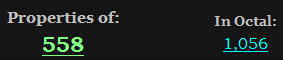156th prime number is 911 a reflection of 119 (God Saturn = 119, All seeing eye = 119, Star of David = 119)
Trump  won the election vote on November Ninth (11/9)
November Ninth = 40+50+700+5+30+2+5+80 + 40+9+40+100+8 = 1109 (Jewish)
November Ninth = 50+60+400+5+40+2+5+90 + 50+9+50+200+8 = 969 (English)
17th tetrahedral number is 969 - the speed of Saturn is 9.69km/s according to our beloved scientism priests
God = 17, EL = 17 (Saturn is known as EL)

This comes on 1/28/2017 - 1+28+2+0+1+7 = 39 - reflection of 93(Saturn) - There's 39 books in the Old Testament that Jews go by, which clearly worships Saturn (EL)
1/28/2017 - 1+2+8+2+0+1+7 = 21 (Saturn = 21 - Reduction)
1/28/2017 - 1+28+20+17 = 66 , Thirty-three = 66(R)/156(Ordinal)The video on this post is 509 seconds long and there will be some 59's coming later in the postThe first line has such nice gematria to open the postTrump's tombstone appeared in Central Park 227 days before he won the election on 314th day in the year, 22/7 = 3.147x7x7 = 343
Twenty-two divided by seven = 314 (Ordinal)
Seven hundred seventy seven = 314 (Ordinal)
Weapons of Mass Destruction = 314 (Ordinal)

Plan on how to defeat ISIS is going to be delivered to Trump 'within 30 days'
Thirty days = 2+8+9+9+2+7 + 4+1+7+10 = 59 (S Exception)
Plan to defeat ISIS = 60+20+1+40 + 100+50 + 4+5+6+5+1+100 + 9+90+9+90 = 590 (Jewish)
Kill = 10+9+20+20 = 59 (Jewish)Michael Flynn = 4+9+3+8+1+5+3 + 6+3+7+5+5 = 59 (Reduced)
Tom Bossert = 2+6+4 + 2+6+1+1+5+9+2 = 38 (Reduced)
Death = 4+5+1+20+8 = 38 (Ordinal)
Killing = 2+9+3+3+9+5+7 = 38 (Reduced)

I think it's going to be very successful = 411 (Ordinal)
Assassin = 1+90+90+1+90+90+9+40 = 411 (Jewish)
They end the post with "That's big stuff"
That's big stuff = 2+8+1+2+1 + 2+9+7 + 1+2+3+6+6 = 50 (Reduced)
Thirty days = 2+8+9+9+2+7 + 4+1+7+1 = 50 (Reduced)
America = 1+13+5+18+9+3+1 = 50 (Ordinal)

## Jan 28, 2017

### CNN - How Donald Trump changed the presidency in 7 days

CNN's post - http://edition.cnn.com/2017/01/27/politics/donald-trump-first-week/index.htmlPayback time = 3+1+2+2+1+3+3 + 7+5+1+5 = 33 (Septenary)
Payback time = 7+1+7+2+1+3+11 + 2+9+4+5 = 52 (K Exception)The video in this post is 2:39 minutes long
Torah = 100+50+80+1+8 = 239 (Jewish)
Golden Gate = 7+50+20+4+5+40 + 7+1+100+5 = 239 (Jewish)

I find this very interesting
Two-three-nine = 2+5+6+2+8+9+5+5+5+9+5+5 = 66 (Reduced)
Two-three-nine = 20+23+15+20+8+18+5+5+14+9+14+5 = 156 (Ordinal)

Thirty-three = 2+8+9+9+2+7+2+8+9+5+5 = 66 (Reduced)
Thirty-three = 20+8+9+18+20+25+20+8+18+5+5 = 156 (Ordinal)How Donald Trump changed the presidency in 7 days = 197 (Reduced)Appropriate headline for the date of release - 1/27/17 = 1+27+17 = 45 - Trump is the 45th term president
seven days = 1+5+4+5+5 + 4+1+7+1 = 33 (Reduced)
This date is a match with Inauguration day as well
1/20/17 - 1+20+17 = 38 (Mr. Trump = 38)
1/27/2017 = 1+27+2+0+1+7 = 38 (Death = 38, Killing = 38), in this post we will see some numbers that go with assassinationsForget the first one hundred days = 6+15+18+7+5+20 + 20+8+5 + 6+9+18+19+20 + 15+14+5 + 8+21+14+4+18+5+4 + 4+1+25+19 = 333 (Ordinal)
Forget the first one hundred days = 6+6+9+7+5+2 + 2+8+5 + 6+9+9+1+2 + 6+5+5 + 8+3+5+4+9+5+4 + 4+1+7+1 = 144 (Reduced)
144th triangular number is 10440
Donald = 4+50+40+1+20+4 = 119 (Jewish)
Mr. Trump = 13+18 + 20+18+21+13+16 = 119 (Ordinal)
Divisors of 119 sum to 14444th prime number is 193.. Forty-four = 144 (Ordinal)
211 is 47th prime number (President = 47 Reduction)Full sentence has 271 gematria (58th prime number... Trump won the 58th presidential election and entered white house with 58 date numerology and so much more)

It doesn't matter what Trump post I start decoding, CNN has been putting the assassination codes all over him, I'm starting to wonder if I am missing something or they are truly constantly pointing to death in May...I'm gonna have to stop decoding Trump, I feel like I'm gonna be showing the same numbers until the day he dies. My prediction for May First still stands.This post is HUGE so I will just go through the subheadingsShattering the norms = 10+8+1+2+2+5+9+9+5+7 + 2+8+5 + 5+6+9+4+10 = 107 (S Exception)
Shattering the norms = 19+8+1+20+20+5+18+9+14+7 + 20+8+5 + 14+15+18+13+19 = 233 (Ordinal)
These 2 numbers coming together is extremely interesting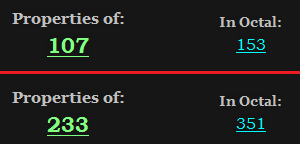28th prime number is 107 - 28 is also 2nd perfect number
233 is 13th Fibonacci number and 51st prime number
First of May = 6+9+9+1+2 + 6+6 + 4+1+7 = 51 (Reduced)
Conspiracy = 3+6+5+1+7+9+9+1+3+7 = 51 (Reduced)

Also he told ABC news that his speech was a "home run"
Home run = 8+15+13+5 + 18+21+14 = 94 (Ordinal)
Ninety-four = 40+9+40+5+100+400+6+50+200+80 = 930 (Jewish)
Divisors of 94 sum to 144
Saturn = 19+1+20+21+18+14 = 93 (Ordinal)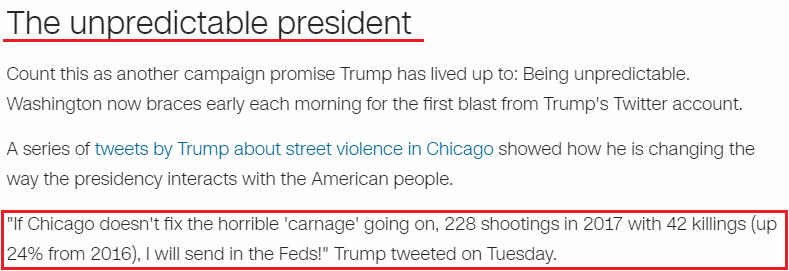The unpredictable president = 100+8+5 + 200+40+60+80+5+4+9+3+100+1+2+20+5 + 60+80+5+90+9+4+5+40+100 = 1035 (Jewish)
The unpredictable president = 20+8+5 + 21+14+16+18+5+4+9+3+20+1+2+12+5 + 16+18+5+19+9+4+5+14+20 = 273 (Ordinal)
These 2 numbers are both very interesting as well
1035 looks a lot like 135 - in my prediction he dies 135 days after start of Saturnalia in 2016

December Seventeenth = 4+5+3+5+4+2+5+9 + 10+5+4+5+5+2+5+5+5+2+8 = 93 (S Exception)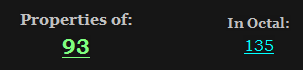135+531 = 666
Also if we measure from Trump's birthday to Saturnalia in 2017 it is 187 days much like the Homicide code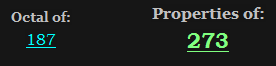The Unpredictable president = 273
Homicide code = 8+15+13+9+3+9+4+5 + 3+15+4+5 = 93 (Ordinal)
one hundred eighty seven = 6+5+5 + 8+3+5+4+9+5+4 + 5+9+7+8+2+7 + 10+5+4+5+5 = 121 (S Exception)He tweeted very interesting numbers, I don't think he even writes his own tweets... he said there was 228 shootings in 2017 with 42 killings which is 24% higher than it was in 2016
carnage = 3+1+80+40+1+7+5 = 137 (Jewish)
33rd prime number is 137

carnage = 3+1+9+5+1+7+5 = 31 (Reduced)
31st prime number is 127, note that they bring this up on 1/27
228 shootings in 2017... of course
death = 24+30+6+120+48 = 228 (Sumerian)
shootings = 90+8+50+50+100+9+40+7+90 = 444 (Jewish)
killing = 66+54+72+72+54+84+42 = 444 (Sumerian)
weapon = 138+30+6+96+90+84 = 444 (Sumerian)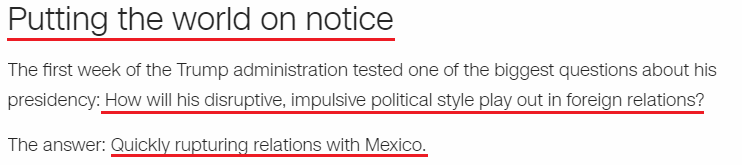Putting the world on notice = 7+3+2+2+9+5+7 + 2+8+5 + 5+6+9+3+4 + 6+5 + 5+6+2+9+3+5 = 118 (Reduced)
Death = 4+5+1+100+8 = 118 (Jewish)
A few days ago I decoded a story that came out on 1/18 full of Trump assassination codes...

Quickly rupturing relations with Mexico. = 187 (Reduced)
Imagine that... 118 followed by homicide code?A restive White House = 1 + 9+5+1+2+9+4+5 + 5+8+9+2+5 + 8+6+3+1+5 = 88 (Reduced)
Trump = 20+18+21+13+16 = 88 (Ordinal)
I won't go into more details with this post since it's one of the bigger ones I've seen

Here are the links to all Trump posts - https://nasanumerology.blogspot.rs/2017/01/artist-protests-trump-with-bloody-mural.html
https://nasanumerology.blogspot.rs/2017/01/cnn-trump-inaguration-howd-he-do.html
https://nasanumerology.blogspot.rs/2017/01/assassination-coding-for-trump-once.html
https://nasanumerology.blogspot.rs/2016/11/possible-assassination-date-for-trump.html